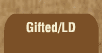Mathematics and Dyslexia

Tips for Learning the Times Tables

Tools for the Times Tables & Workbook

Home > Math > times tables practice > Sevens (low, scrambled)

## The Sevens Times Tables

No easy tricks for the sevens... though you should remember that because it's an odd number, the answer will be even if you multiply by an even number odd if you multiply by odd.

1. 7 x 3=
2. 7 x 5=
3. 7 x 1=
4. 3 x 7=
5. 7 x 5=
6. 4 x 7=
7. 7 x 2=
8. 2 x 7=
9. 3 x 7=
10. 7 x 4=
11. 0 x 7=
12. 7 x 5=
13. 7 x 4=
14. 7 x 2=
15. 7 x 3=